Electron. J. Diff. Eqns., Vol. 2003(2003), No. 66, pp. 1-11.

### Life span of nonnegative solutions to certain quasilinear parabolic Cauchy problems Hendrik J. Kuiper

Abstract:
We consider the problem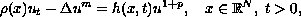with nonnegative, nontrivial, continuous initial condition,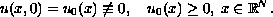An integral inequality is obtained that can be used to find an exponent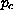such that this problem has no nontrivial global solution when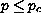. This integral inequality may also be used to estimate the maximal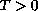such that there is a solution for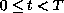. This is illustrated for the case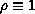and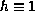with initial condition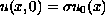,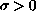, by obtaining a bound of the form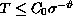.

Submitted May 15, 2003. Published June 13, 2003.
Math Subject Classifications: 35K55, 35B33, 35B30.
Key Words: Nonlinear parabolic equation, blow-up, lifespan, critical exponent.

Show me the PDF file (236K), TEX file, and other files for this article.Hendrik J. Kuiper Department of Mathematics Arizona State University Tempe, AZ 85287-1804 USA email: kuiper@asu.edu